## Filters

Sort by :
Clear All
Q
Ideal gas equation, PV = nRT n = w/M where w is weight of gas and M is molar mass PV = w/M (RT) => PM = w/V (RT)                    (density, d =w/V) => PM = dRT d = PM/RT d = 5x28/0.082x500 = 3.41 g/ml
Alpha emission :  Beta Emission :  In this problem, both alpha and beta emissions are taking place. Putting value of A and Z Now in the product nucleus, Number of protons = 82 and number of neutrons = 206-82 =  124 Ratio of Neutron/Proton = 124/82 = 62/41
Given, V =nU Mirror formula,  In concave mirror
No potential energy is not negative when you displace particle against gravitation.  Take help of vectors for understanding this, Now if you are displacing particle against gravity that means you are taking particle in upward direction while the direction of g is in downward. So the dot product will give positive value equal to mgh.
Vapour pressure of liquid ‘S’ at 500 K is approximately equal to
As we have learnt,   Δ G of equilibrium -   - wherein At Equilibrum     and
Work done by frictional is zero when the object to which it is acing does not move any distance.

A ray of light is incident on a medium with angle of incidence i and refracted into a second medium with angle of refreaction r. The graph of sin i vs sin r is shown in the figures. Then the velocity of light in the first medium is n times the velocity of light in the second medium. The value of n should be.

As the figure is not mentioned, I would be suggesting a way to solve this problem. Now, Speed of the light in that medium  can be told with the help of the Refractive Index.         Refractive Index = Sini/Sinr where i is the angle of Incidence and r is the angle of the Refraction.  But, Refractive Index = Speed of light in medium 1/Speed of light in medium 2.  ∴ Speed of light in medium...
Dimension of Energy = Dimension of Work W = F.l If units of force and length increases 4 times then, W = 4F.4l = 16F.l  Hence unit of Energy will increase 16 times

Equation of sound wave in air is given by. Find the speed of sound in air.

Compare with general equation   Pressure Waves -   - wherein  = Maximum variation in pressure
In an electrical circuit R, L, C and an a.c. voltage source are all connected in series. When L is removed from the circuit, the phase difference between the voltage and the current in the circuit is pi/3 . If instead, C is removed from the circuit, the phase difference is again pi/3 . The power factor of the circuit is:
As we learnt in Impedence - -     Power factor -   - wherein resistance impedence              when C is removed from the Circuit Power factor
Engineering
762 Views   |

A physical quantity P is described by the relation

P= a1/2 b2 c3 d-4

If the relative errors in the measurement of a, b, c and d respectively, are 2%, 1%, 3% and 5%,

then the relative error in P will be :

• Option 1)

8%

• Option 2)

12%

• Option 3)

32%

• Option 4)

25%

P = a1/2b2C3d-4100() =  =  Option 1) 8% this is incorrect option Option 2) 12% this is incorrect option Option 3) 32% this is correct option Option 4) 25% this is incorrect option
Engineering
100 Views   |

A standing wave is formed by the superposition of two waves travelling in opposite directions.  The transverse displacement is given byWhat is the speed of the travelling wave moving in the positive x direction ?

(x and t are in meter and second, respectively.)

• Option 1)

160 m/s

• Option 2)

90 m/s

• Option 3)

180 m/s

• Option 4)

120 m/s

As discussed in @7297 Speed of the travelling wave in the X direction will be 160 ms-1 Option 1)  160 m/s This option is correct Option 2) 90 m/s This option is incorrect Option 3) 180 m/s This option is incorrect Option 4)  120 m/s   This option is incorrect
Engineering
869 Views   |

In a meter bridge experiment resistance are connected as shown in the figure. Initially resistance P=4 and the neutral point N is at 60 cm from A.  Now an unknown resistance R is connected in series to P and the new position of the neutral point is at 80 cm from A.  The value of unknown resistance R is :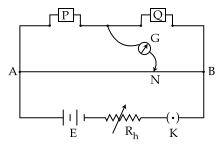• Option 1)• Option 2)• Option 3)• Option 4)As we learnt in  To find resistance of a given wire using metre bridge and hence determine the specific resistance - For unknown resistance x= Unknown resistance  R= Given resistance   - wherein Calculation = .........ohms  = Resistivity X = Unknown resistance D = Diametre L = length of wire               Option 1)         This option is incorrect Option 2) This option is...
Engineering
702 Views   |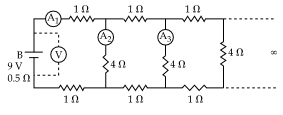A 9 V battery with internal resistance of 0.5 is connected across an infinite network as shown in the figure.  All ammeters A1, A2, A3 and voltmeter V are ideal.

Choose correct statement.

• Option 1)

Reading of A1 is 2 A

• Option 2)

Reading of A1 is 18 A

• Option 3)

Reading of V is 9 V

• Option 4)

Reading of V is 7 V

As we learnt in  To determine resistance of given wire by plotting graph between V versus I - V= IR R= Resistance I = Current v= Voltage - wherein   Procedure:  1.    Make circuit diagram as shown in the figure and arrange all the apparatus according to it. 2.    Clean the ends of the connecting wires with the help of sand paper.  3.    Find out the least counts of voltmeter and ammeter...
Engineering
240 Views   |

### Which of the following statements is false ?

• Option 1)

Wheatstone bridge is the most sensitive when all the four resistances are of the same order of magnitude.

• Option 2)

In a balanced wheatstone bridge if the cell and the galvanometer are exchanged, the null point is disturbed.

• Option 3)

A rheostat can be used as a potential divider.

• Option 4)

Kirchhoff’s second law represents energy conservation.

As we learnt in

To find resistance of a given wire using metre bridge and hence determine the specific resistance -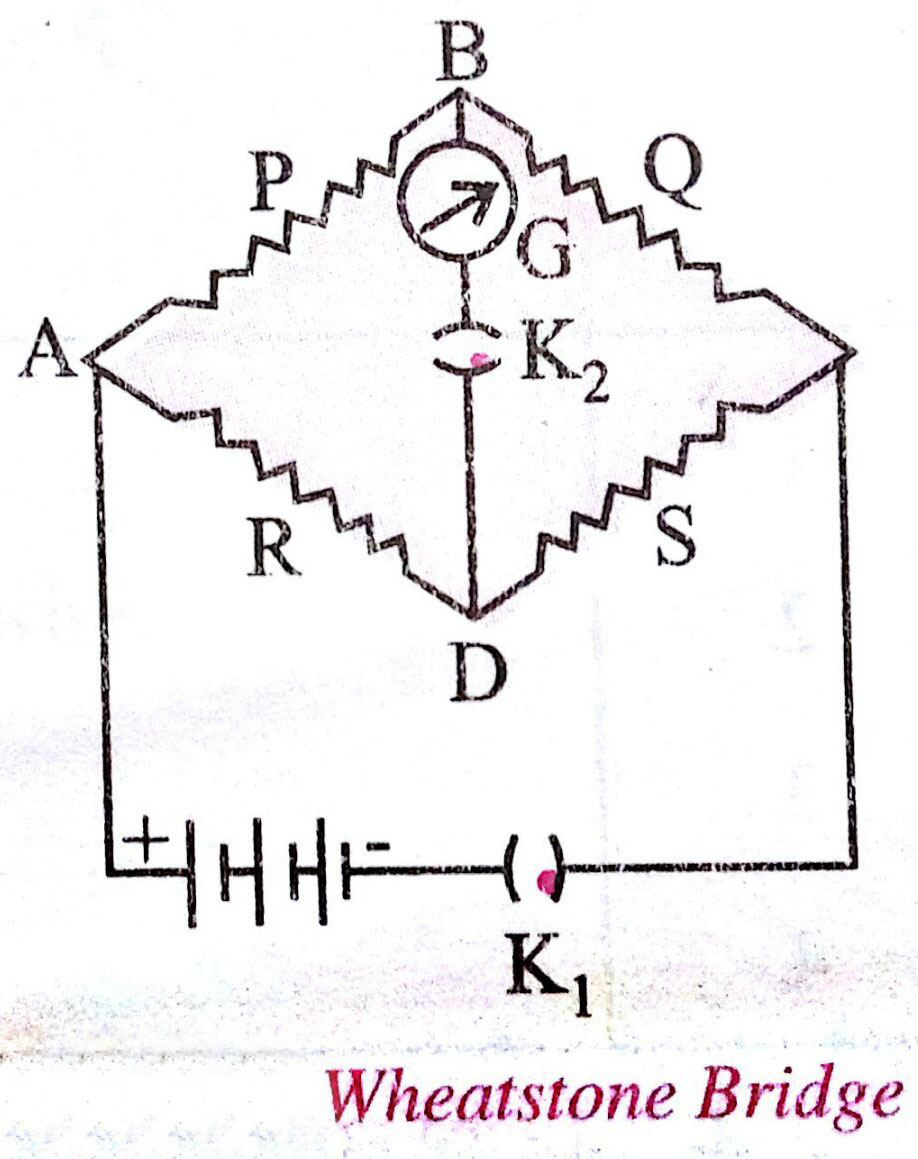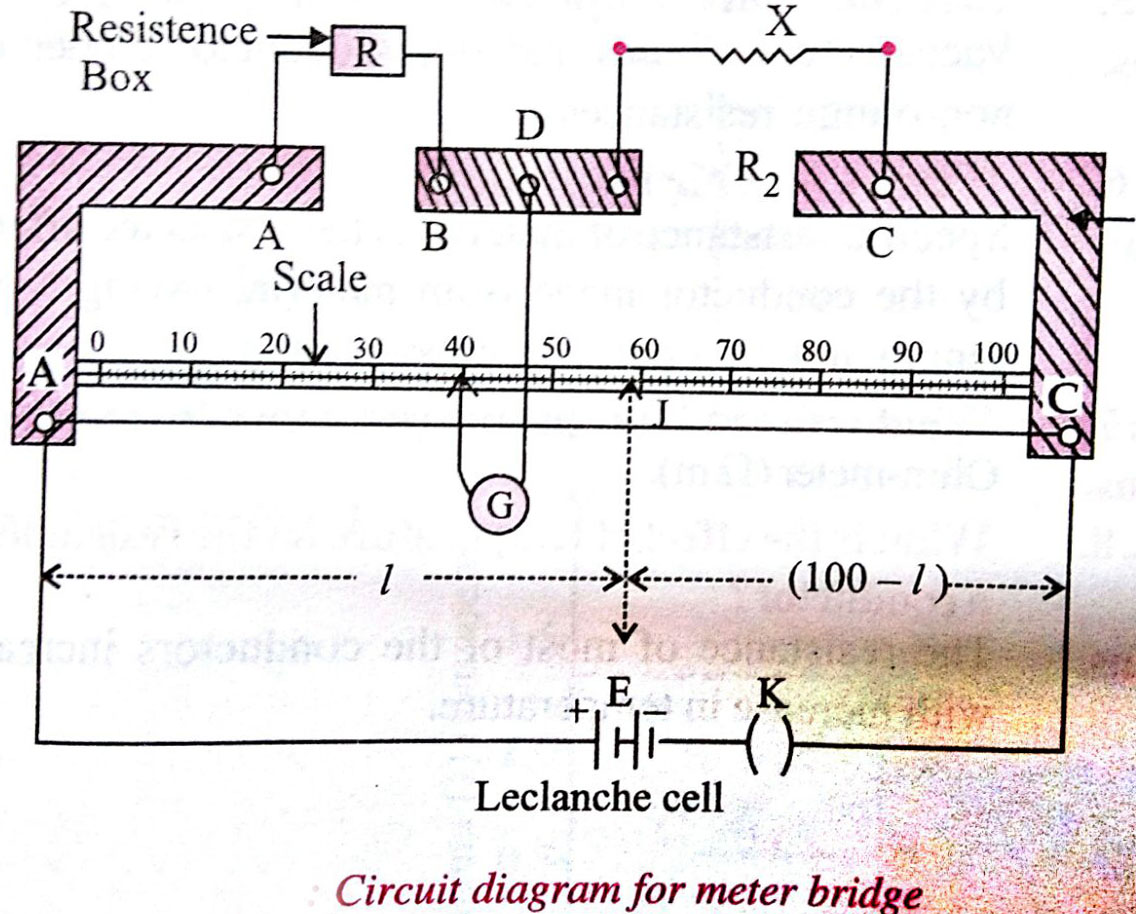For unknown resistance

x= Unknown resistance

R= Given resistance

- wherein

Calculation

= .........ohms

= Resistivity

X = Unknown resistance

D = Diametre

L = length of wire

There is no change in null point, if the cell and the galvanometer are exchanged in a balanced bridge.

So, option (2) is the answer

Balancing condition

Balancing condition

Option 1)

Wheatstone bridge is the most sensitive when all the four resistances are of the same order of magnitude.

Incorrect

Option 2)

In a balanced wheatstone bridge if the cell and the galvanometer are exchanged, the null point is disturbed.

Correct

Option 3)

A rheostat can be used as a potential divider.

Incorrect

Option 4)

Kirchhoff’s second law represents energy conservation.

Incorrect

View More
Engineering
625 Views   |

A magnetic needle of magnetic moment 6.7×10−2 Am2 and moment of inertia 7.5×10−6 kg m2 is performing simple harmonic oscillations in a magnetic field of 0.01 T.  Time taken for 10 complete oscillations is :

• Option 1)

6.65 s

• Option 2)

8.89 s

• Option 3)

6.98 s

• Option 4)

8.76 s

As we learnt in Time period of Oscillating Bar Magnet - - wherein I = Moment of Inertia of short bar magnet  M - Mass of bar magnet         Hence T = 6.65 s   Option 1)  6.65 s   Correct Option 2) 8.89 s   Incorrect  Option 3)  6.98 s   Incorrect  Option 4)  8.76 s Incorrect
Engineering
404 Views   |

A magnetic dipole in a constant magnetic field has :

• Option 1)

maximum potential energy when the torque is maximum.

• Option 2)

zero potential energy when the torque is minimum.

• Option 3)

zero potential energy when the torque is maximum.

• Option 4)

minimum potential energy when the torque is maximum.

As we learnt in Torque - T=   - wherein M - magnetic moment      Work done by current carrying coil - -    For maximum torque  and for this value of  potential energy will be zero.. Correct option is 3.     Option 1) maximum potential energy when the torque is maximum. Incorrect Option 2)  zero potential energy when the torque is minimum. Incorrect Option 3)  zero potential energy when the...
Engineering
1339 Views   |

A negative test charge is moving near a long straight wire carrying a current.  The force acting on the test charge is parallel to the direction of the current.  The motion of the charge is :

• Option 1)

away from the wire

• Option 2)

towards the wire

• Option 3)

parallel to the wire along the current

• Option 4)

parallel to the wire opposite to the current

As we learnt in Fleming's left hand rule - Direction of force on charged particle in magnetic field can also be found by fleming's left hand rule  - wherein     According to fleming's left hand rule the direction of motion of charge is towards the wire. Option 1)  away from the wire This is incorrect option. Option 2)  towards the wire This is correct option. Option 3)  parallel to the wire...
Engineering
939 Views   |

A uniform magnetic field B of 0.3 T is along the positive Z-direction.  A rectangular loop (abcd) of sides 10 cm×5 cm carries a current I of 12 A.  Out of the following different orientations which one corresponds to stable equilibrium ?

• Option 1)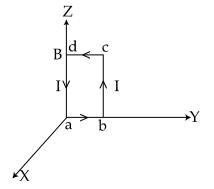• Option 2)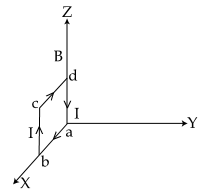• Option 3)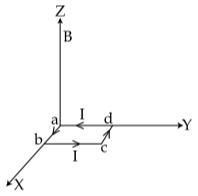• Option 4)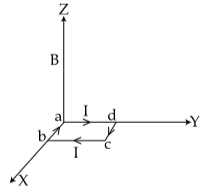As we learnt in  Magnetic moment (M) - M=NiA - wherein N-number of turns in the coil  i-current throughout the coil  A-area of the coil     and      Current loop as a magnetic dipole - A current carrying circular coil behaves as a bar magnet whose magnetic monent is M - wherein    Magnetic moment of current carrying rectangular loop of Area A is given by M= NiA   Magnetic moment of current...
Engineering
391 Views   |

In a certain region static electric and magnetic fields exist.  The magnetic field is given by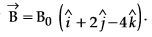If a test charge moving with a velocity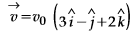experiences no force in that region, then the electric field in the region, in SI units, is :

• Option 1)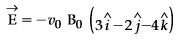• Option 2)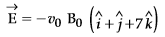• Option 3)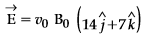• Option 4)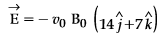As we learnt in Magnetic field If V(vector), E (vector) and B (vector) are mutually perpendicular - Fe=Fm -     Electric field produced by the charge Option 1) This is incorrect option. Option 2) This is incorrect option. Option 3) This is incorrect option. Option 4) This is correct option.
Exams
Articles
Questions